#2017 iT 邦幫忙鐵人賽
DAY 9
1
Big Data

• 基本型別
• 檢查型別函式
• 轉換函式
• NA處理

## R語言基本資料型別

• 字元(character): 文字字串，用雙引號包起來。
• 數值(numeric): 雙倍精準度數值，簡單的形容就是double。
• 整數(integer): 沒有小數位的數值。
• 複數(complex): 虛數，實際還沒用過。
• 邏輯(logical): TRUE /FALSE，也可以縮寫成T/F。
• 資料列: 很少使用到。

• 日期時間: POSIXct、POSIXt

## 變數的指派

• = (R Manual有提到有些地方，沒辦法適用)
• <- 最佳
• -> (也有右向箭頭，但不建議，習慣一種就好)

http://stat.ethz.ch/R-manual/R-patched/library/base/html/assignOps.html

## 自動型別推斷(type inference)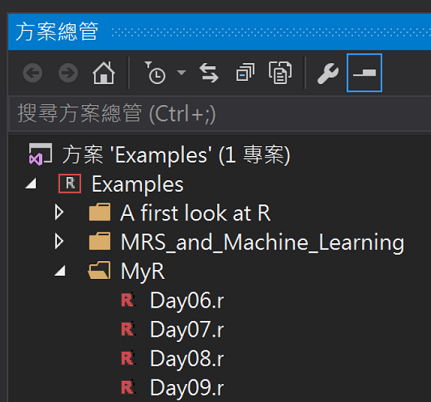#### 字元

``````v <- "正妹身高"
print(class(v))
print(typeof(v))
``````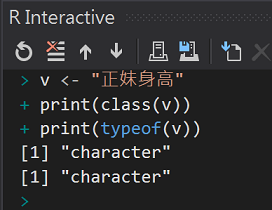#### 數值

``````v <- 160.5
print(class(v))
print(typeof(v))
``````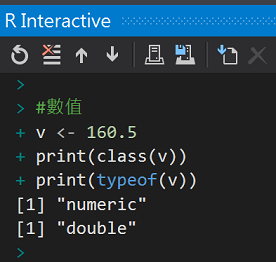#### 整數

``````v <- 47L
print(class(v))
print(typeof(v))
``````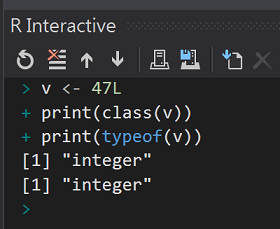#### 複數

``````v <- 1+2i
print(class(v))
print(typeof(v))
``````#### 邏輯

``````v <- TRUE
print(class(v))
print(typeof(v))
``````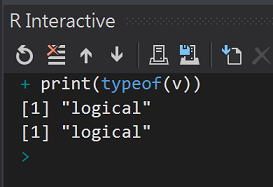#### 資料列

``````v <- charToRaw("Hello")
print(class(v))
print(typeof(v))
``````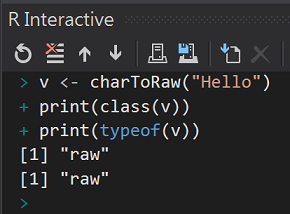#### 日期 POSIXct、POSIXt

``````v <- Sys.time()
print(class(v))
print(typeof(v))
``````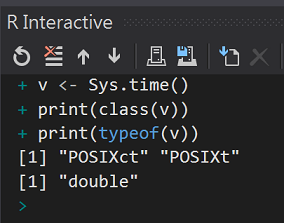• 有雙引號的為字元
• 看起來像數值，但沒有L標示的是雙倍精準度數值
• 看起來像數值，但標示L為整數數值。
• 有i(虛數)節尾的數值，推斷為複數
• TRUE/FALSE或T/F保留字判定為logical

## 檢查資料是否為指定型別

• is.character(v)
• is.numeric(v)
• is.integer(v)
• is.complex(v)
• is.logical(v)
• is.na(v)
• isDate(v)

#### 字元

``````print("字元結果:")
is.character(1)
is.character("1")
``````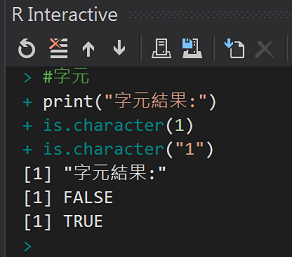`""`引號重要！

#### 數值

``````print("數值結果:")
is.numeric(1)
is.numeric("1")
``````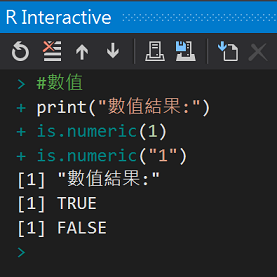#### 整數

``````print("整數結果:")
is.integer(3.14)
is.integer(3)
is.integer(as.integer(3))
``````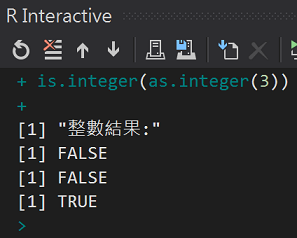#### 邏輯

``````print("邏輯結果:")
is.logical(TRUE)
is.logical(T)
is.logical("TRUE")
``````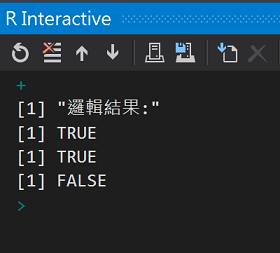#### 是否為null

``````is.na(NA)
is.na("")
``````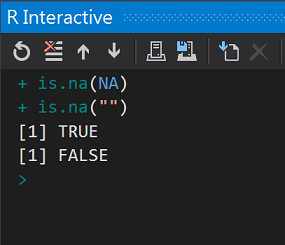#### 日期，手作一個

``````isDate <- function(x) {
tryCatch(!is.na(as.Date(x, date.format = "%Y/%m/%d")),
error = function(err) {
FALSE
})
}

isDate("2016/12/31")
isDate("2016/12/32")
``````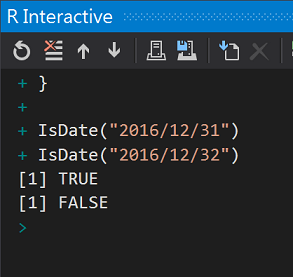## 型別間轉換函式

#### 轉換為字串

``````as.character(3.14)
as.character(1)
as.character(TRUE)
``````#### 轉換為數值

``````as.numeric("-3.14")
as.numeric("-3")
as.numeric(TRUE)
as.numeric("3點14")
``````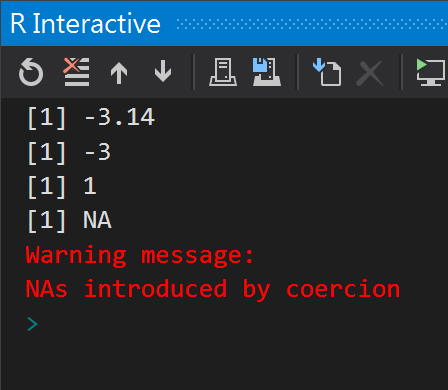`TRUE`轉換為1，最後一個`"3點14"`轉換失敗，`回傳NA`

#### 轉換為整數

``````as.integer("-3.14")
as.integer("-3")
as.integer(TRUE)
as.integer("3點14")
``````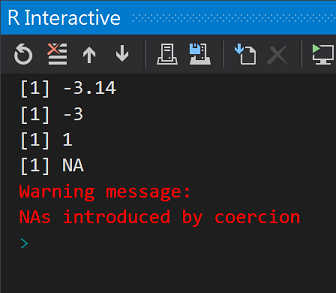#### 轉換為日期

``````as.Date("2016/12/31", date.format = "%Y/%m/%d")
as.Date("2016/02/30", date.format = "%Y/%m/%d")
``````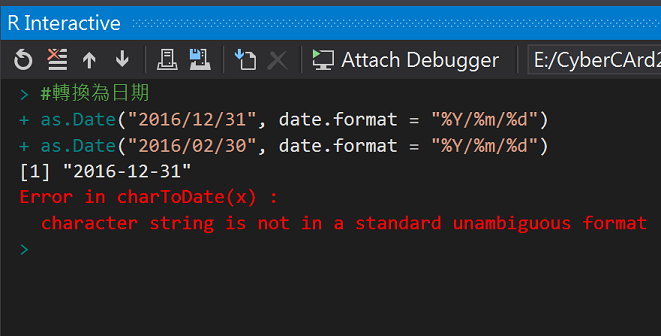## NA狀態處理

R語言中的Null值(空值)是以`NA`表示，Null代表著物件值的狀態，不能作為運算。

``````is.na(NA)
#轉換失敗時拋回NA
x <- as.numeric("3點14")
x
is.na(NA)
``````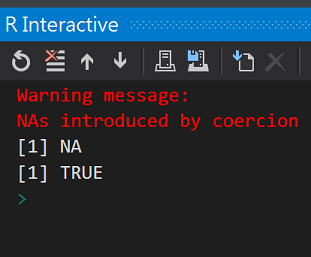## 資料型別名詞

R語言的資料型別名詞:

• Class
• Type
• Mode
• storage.mode

• Class比較像程式執行時識別封裝後變數使用的
• type和stroage.mode則是接近儲存時的型態
• 為了和S語言相容多了mode:

.NET/Java世界中，Class是藍圖，實體則通常是物件object。

#### 查詢內建資料集

``````data()
``````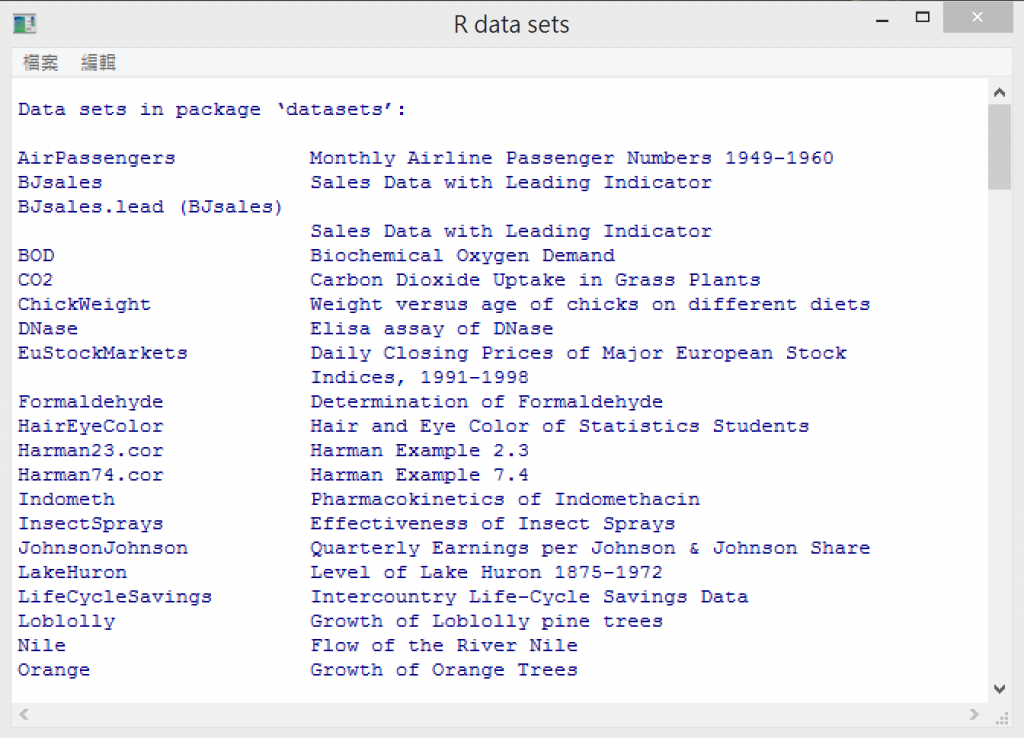R語言`datasets` package中有內建的資料集，有時安裝新的Package後(`rpart``rMaps``dplyr`..)，也會有練習用的資料集。

``````v1 <- HairEyeColor
print(class(v1))
print(typeof(v1))
print(mode(v1))
print(storage.mode(v1))

print("---我是分隔線----")

v2 <- cars
print(class(v2))
print(typeof(v2))
print(mode(v2))
print(storage.mode(v2))
``````

Variable Explorer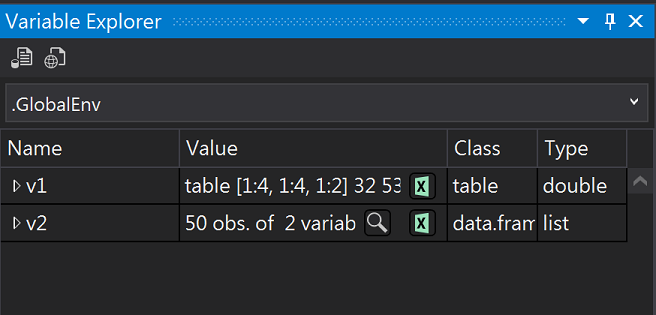R語言與機器學習見面會30